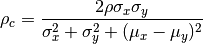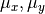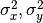Shortcuts

# Concordance Corr. Coef.¶

## Module Interface¶

class torchmetrics.ConcordanceCorrCoef(num_outputs=1, **kwargs)[source]

Computes concordance correlation coefficient that measures the agreement between two variables. It is defined as.whereis the means for the two variables,are the corresponding variances and rho is the pearson correlation coefficient between the two variables.

As input to forward and update the metric accepts the following input:

• preds (Tensor): either single output float tensor with shape (N,) or multioutput float tensor of shape (N,d)

• target (Tensor): either single output float tensor with shape (N,) or multioutput float tensor of shape (N,d)

As output of forward and compute the metric returns the following output:

• concordance (Tensor): A scalar float tensor with the concordance coefficient(s) for non-multioutput input or a float tensor with shape (d,) for multioutput input

Parameters
Example (single output regression):
>>> from torchmetrics import ConcordanceCorrCoef
>>> import torch
>>> target = torch.tensor([3, -0.5, 2, 7])
>>> preds = torch.tensor([2.5, 0.0, 2, 8])
>>> concordance = ConcordanceCorrCoef()
>>> concordance(preds, target)
tensor(0.9777)

Example (multi output regression):
>>> from torchmetrics import ConcordanceCorrCoef
>>> import torch
>>> target = torch.tensor([[3, -0.5], [2, 7]])
>>> preds = torch.tensor([[2.5, 0.0], [2, 8]])
>>> concordance = ConcordanceCorrCoef(num_outputs=2)
>>> concordance(preds, target)
tensor([0.7273, 0.9887])


Initializes internal Module state, shared by both nn.Module and ScriptModule.

## Functional Interface¶

torchmetrics.functional.concordance_corrcoef(preds, target)[source]

Computes concordance correlation coefficient that measures the agreement between two variables. It is defined as.whereis the means for the two variables,are the corresponding variances and rho is the pearson correlation coefficient between the two variables.

Parameters
Example (single output regression):
>>> from torchmetrics.functional import concordance_corrcoef
>>> target = torch.tensor([3, -0.5, 2, 7])
>>> preds = torch.tensor([2.5, 0.0, 2, 8])
>>> concordance_corrcoef(preds, target)
tensor([0.9777])

Example (multi output regression):
>>> from torchmetrics.functional import concordance_corrcoef
>>> target = torch.tensor([[3, -0.5], [2, 7]])
>>> preds = torch.tensor([[2.5, 0.0], [2, 8]])
>>> concordance_corrcoef(preds, target)
tensor([0.7273, 0.9887])

Return type

Tensor

© Copyright Copyright (c) 2020-2023, Lightning-AI et al... Revision 825d17f3.

Built with Sphinx using a theme provided by Read the Docs.
Versions
latest
stable
v0.11.3
v0.11.2
v0.11.1
v0.11.0
v0.10.3
v0.10.2
v0.10.1
v0.10.0
v0.9.3
v0.9.2
v0.9.1
v0.9.0
v0.8.2
v0.8.1
v0.8.0
v0.7.3
v0.7.2
v0.7.1
v0.7.0
v0.6.2
v0.6.1
v0.6.0
v0.5.1
v0.5.0
v0.4.1
v0.4.0
v0.3.2
v0.3.1
v0.3.0
v0.2.0
v0.1.0